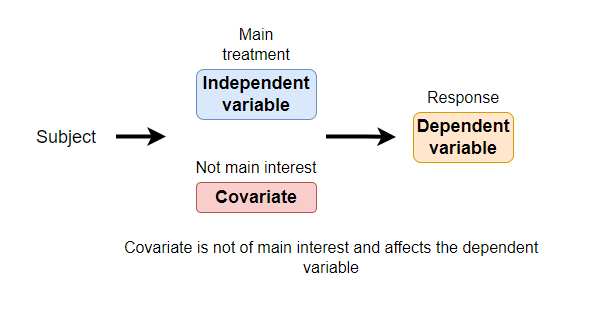# Covariate in Statistics: Definition and Examples

## What is Covariate?

In statistical experiments, researchers often measure the independent variable (main treatment variable) and the dependent variable (response to treatment).

Additionally, there could be other variables known to affect the dependent variable besides the main treatment variable. Generally, this variable is not of main interest in the experiment and is referred to as a covariate.

Definition: Covariate is a variable that is not of main interest in an experiment but can affect the dependent variable and the relationship of the independent variable with the dependent variable.The covariate is not a planned variable but often arises in experiments due to underlying experimental conditions.

Covariate should be identified and analyzed to increase the accuracy and reduce the unexplained variation of the statistical model. Hence, the covariate is also known as a control variable.

## Covariate example

For example, a plant researcher wants to test whether the yield of the plant depends on the genotype of the plant. The researcher collects the data for a yield of the different plant genotypes.

However, the researcher also knows that the height of the plants also affects the plant yield. The plant height is not of primary interest to the researcher, but it should be considered in a statistical model to get accurate results.

In this example, plant genotype is an independent variable (main treatment variable), plant yield is a dependent variable (response variable), and plant height is a covariate.

Note: Covariate is always a continuous variable

## Covariate in Statistical analysis

The ANCOVA (Analysis of Covariance) and regression analysis are commonly used statistical methods that consider covariate in the model.

### ANCOVA (Analysis of Covariance)

ANCOVA is an extension to ANOVA in which a covariate is considered in the statistical model.

The ANOCVA analyzes the effect of the independent variable (main treatment variable) on the dependent variable while there is a covariate in the study.

In the example discussed above, the researcher could have used one-way ANOVA to study the effect of plant genotypes on yield. But these results could be misleading without considering the effect of plant height (covariate) on plant yield.

ANCOVA considers the covariate in the model and estimates the differences in genotypes while adjusting the effect of plant height (covariate). ANCOVA increases the accuracy of the model by removing the variance associated with the covariate.

### Regression analysis

Simple and multiple regression analyses are useful for studying the relationship between independent variables and a dependent variable.

The simple regression analysis is used for understanding the relationship between one independent variable with that of the dependent variable. Whereas, multiple regression analysis is used for understanding the relationship between multiple independent variables with that of the dependent variable.

For example, the effect of plant height on plant yield can be quantified using simple regression analysis.

In addition to plant height, the researcher also knows that ambient temperature also affects the yield of the plant. In this case, the ambient temperature could be used as a covariate in the model.

The multiple regression analysis can be performed in between plant height and ambient temperature as independent variables and plant yield as a dependent variable.

The multiple regression analysis reports adjusted R-squared and regression coefficients (slope), which can be used for estimating the effect of plant height on plant yield after adjusting the effect for ambient temperature.

Note: Sometimes in regression analysis, the independent variables are also known as covariates. This is because they predict the outcome of the dependent variable and can be of primary interest.